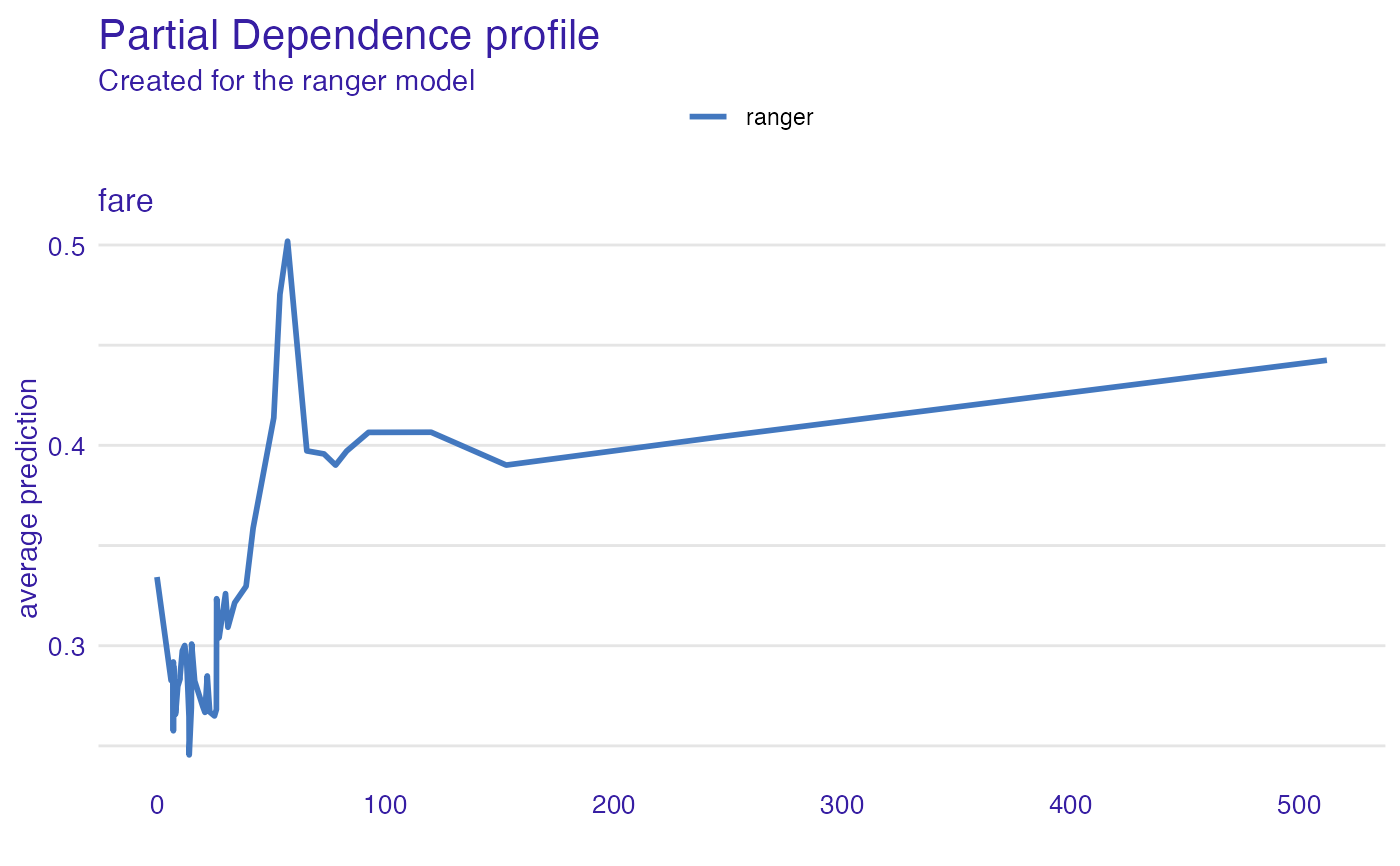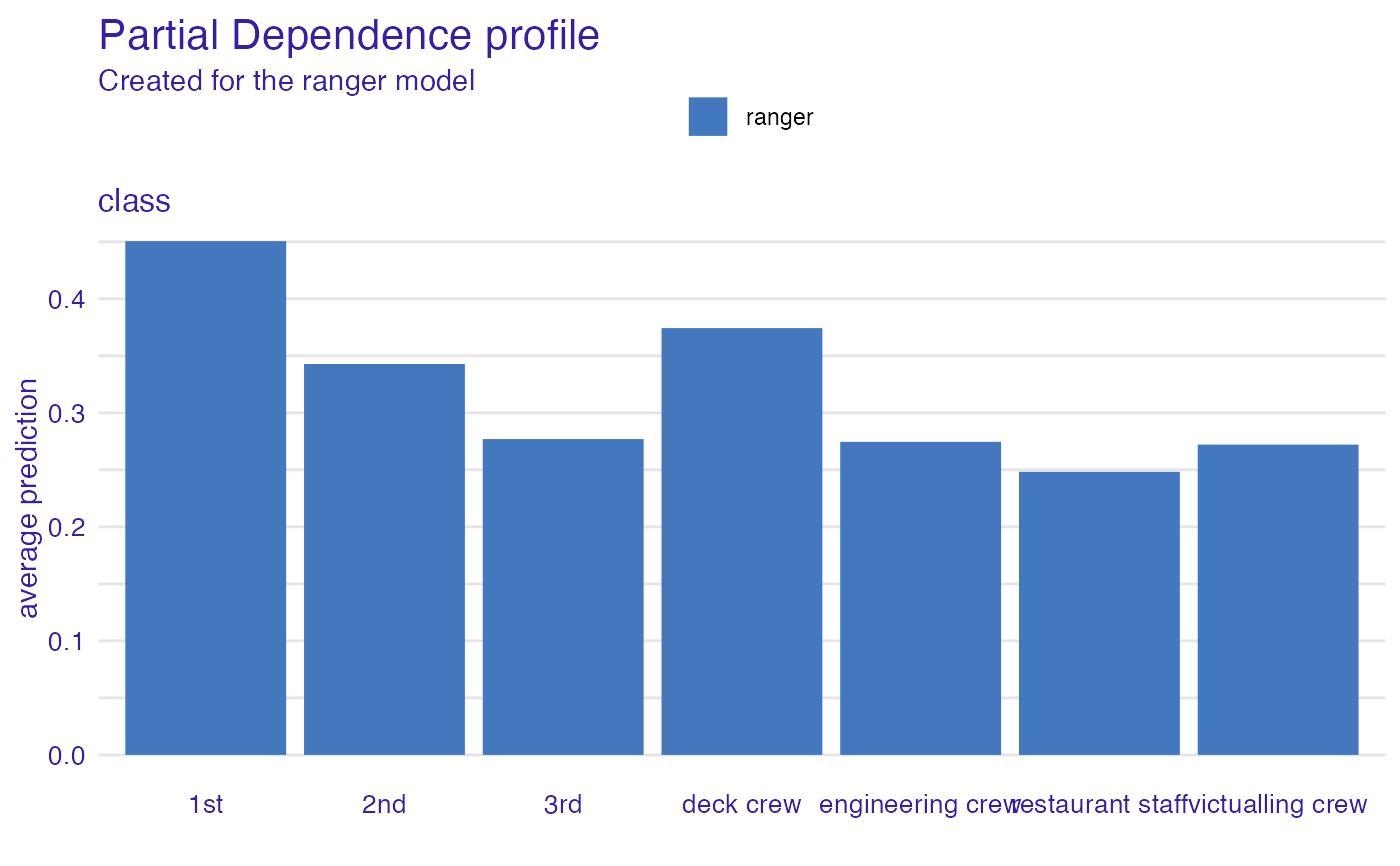From DALEX version 1.0 this function calls the accumulated_dependence or partial_dependence from the ingredients package. Find information how to use this function here: https://ema.drwhy.ai/partialDependenceProfiles.html.

variable_effect(explainer, variables, ..., type = "partial_dependency")

variable_effect_partial_dependency(explainer, variables, ...)

variable_effect_accumulated_dependency(explainer, variables, ...)

## Arguments

explainer

a model to be explained, preprocessed by the 'explain' function

variables

character - names of variables to be explained

...

other parameters

type

character - type of the response to be calculated. Currently following options are implemented: 'partial_dependency' for Partial Dependency and 'accumulated_dependency' for Accumulated Local Effects

## Value

An object of the class 'aggregated_profiles_explainer'. It's a data frame with calculated average response.

## Examples

titanic_glm_model <- glm(survived~., data = titanic_imputed, family = "binomial")
explainer_glm <- explain(titanic_glm_model, data = titanic_imputed)
#> Preparation of a new explainer is initiated
#>   -> model label       :  lm  (  default  )
#>   -> data              :  2207  rows  8  cols
#>   -> target variable   :  not specified! (  WARNING  )
#>   -> predict function  :  yhat.glm  will be used (  default  )
#>   -> predicted values  :  No value for predict function target column. (  default  )
#>   -> model_info        :  package stats , ver. 4.2.0 , task classification (  default  )
#>   -> model_info        :  Model info detected classification task but 'y' is a NULL .  (  WARNING  )
#>   -> model_info        :  By deafult classification tasks supports only numercical 'y' parameter.
#>   -> model_info        :  Consider changing to numerical vector with 0 and 1 values.
#>   -> model_info        :  Otherwise I will not be able to calculate residuals or loss function.
#>   -> predicted values  :  numerical, min =  0.008128381 , mean =  0.3221568 , max =  0.9731431
#>   -> residual function :  difference between y and yhat (  default  )
#>   A new explainer has been created!
expl_glm <- variable_effect(explainer_glm, "fare", "partial_dependency")
#> Warning: 'variable_effect()' is deprecated. Use 'DALEX::model_profile()' instead.
#> Find examples and detailed introduction at: https://pbiecek.github.io/ema/
plot(expl_glm)# \donttest{
library("ranger")
titanic_ranger_model <- ranger(survived~., data = titanic_imputed, num.trees = 50,
probability = TRUE)
explainer_ranger  <- explain(titanic_ranger_model, data = titanic_imputed)
#> Preparation of a new explainer is initiated
#>   -> model label       :  ranger  (  default  )
#>   -> data              :  2207  rows  8  cols
#>   -> target variable   :  not specified! (  WARNING  )
#>   -> predict function  :  yhat.ranger  will be used (  default  )
#>   -> predicted values  :  No value for predict function target column. (  default  )
#>   -> model_info        :  package ranger , ver. 0.13.1 , task classification (  default  )
#>   -> model_info        :  Model info detected classification task but 'y' is a NULL .  (  WARNING  )
#>   -> model_info        :  By deafult classification tasks supports only numercical 'y' parameter.
#>   -> model_info        :  Consider changing to numerical vector with 0 and 1 values.
#>   -> model_info        :  Otherwise I will not be able to calculate residuals or loss function.
#>   -> predicted values  :  numerical, min =  0.008905977 , mean =  0.3227188 , max =  0.9978063
#>   -> residual function :  difference between y and yhat (  default  )
#>   A new explainer has been created!
expl_ranger  <- variable_effect(explainer_ranger, variables = "fare",
type = "partial_dependency")
plot(expl_ranger)plot(expl_ranger, expl_glm)# Example for factor variable (with factorMerger)
expl_ranger_factor  <- variable_effect(explainer_ranger, variables =  "class")
#> 'variable_type' changed to 'categorical' due to lack of numerical variables.
plot(expl_ranger_factor)# }